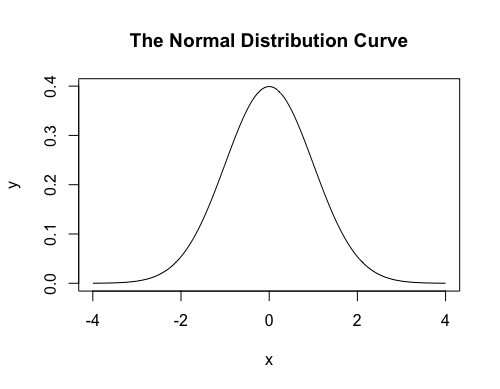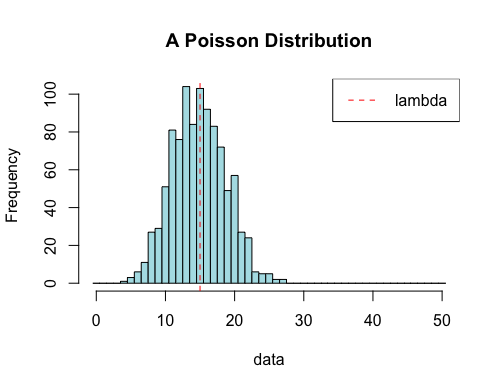Making Plots in R

1. Write an R function binomial.hist(n,p) that when invoked creates a histogram of the results of 1000 binomial experiments of $n$ trials with probability of success $p$. Each class (or "bin") should be 1 unit wide and centered on an integer number of successes. Given there are $n$ trials, the maximum $x$ value should then be $n+0.5$.

As sample output, the below figure shows one possible outcome of binomial.hist(30,0.7). Of course, the exact heights of the rectangles involved will vary slightly from one simulation to the next.binomial.hist = function(n,p) {
data = rbinom(1000,size=n,prob=p)
hist(data,breaks=seq(from=-0.5,to=(n+0.5),by=1))
}

2. Write an R function normal.curve.plot() that when invoked draws the graph of the following function from $x=-4$ to $x=4$, as shown below. Make sure that your graph is titled "The Normal Distribution Curve", and the $x$ and $y$ axes are labeled $x$ and $y$, respectively. (Hints: the function exp(x) computes $e^x$, and the constant pi has value $\pi$.) $$f(x) = \frac{e^{\frac{-x^2}{2}}}{\sqrt{2\pi}}$$normal.curve.plot = function() {
xs = seq(from=-4,to=4,by=0.01)
ys = exp(-xs^2/2)/sqrt(2*pi)
plot(xs,ys,type="l",xlab="x",ylab="y",main="The Normal Distribution Curve")
}

3. Write an R function poisson.plot(n, lambda, max.occurrences.displayed) that simulates $n$ outcomes of a random variable that follows a Poisson distribution with an expected number of occurrences equal to lambda and displays a related histogram. The max.occurrences.displayed argument should specify the largest integer value of $x$ that appears in your plot (since there is some non-zero probability any positive integer number of occurrences could occur). Any simulated data values greater than max.occurrences.displayed should be discarded before attempting to create the histogram.

As sample output, consider the results of one call to poisson.plot(1000,15,50), shown below. Note, the bars of the histogram should not be white (pick your favorite color), a dotted, red vertical line should mark the position of $\lambda$, and a legend should be included on the graph noting the same.poisson.plot = function(n,lambda,max.occurrences.displayed) {
data = rpois(n,lambda)
data = data[data <= max.occurrences.displayed]
hist(data,breaks=seq(from=-0.5,to=(max.occurrences.displayed+0.5),by=1),
main="A Poisson Distribution",col="powderblue")
abline(v=lambda,lty=2,col="red")
legend("topright",
legend=c("lambda"),
lty=c(2),
col=c("red"),
lwd=c(1))
}

4. Write an R function chebyshev(k) that will plot 30 points, whose $x$-coordinates are randomly and uniformly distributed between $0$ and $1$, and whose $y$-coordinates are all equal to $1$. A small blue vertical line should mark the mean $x$ value, with small (but slightly larger) red dashed vertical lines marking the positions that are $k$ standard deviations from the mean. Text should be displayed in a slightly smaller font than the default, and above the $30$ points, that indicates 1) the value of $k$ with which the function was invoked; 2) the proportion of points whose $x$ value fell within $k$ standard deviations from the mean (i.e., inside the red "fences"); and 3) the minimum associated proportion guaranteed by Chebyshev's Theorem. The numerical values in the text displayed should be rounded, as necessary, to 4 digits past the decimal point. (Consider using the round() function.) The title of the plot should be "Demonstration of Chebyshev's Theorem", and the $x$ and $y$ axes should have no labels shown.

As sample output, consider the results of one call to chebyshev(1.1), shown belowchebyshev = function(k) {
xs = c(runif(30))
ys = rep(1,times=30)
plot(xs,ys,main="Demonstration of Chebyshev's Theorem",xlab="",ylab="")
lower.bound = mean(xs) - k*sd(xs)
upper.bound = mean(xs) + k*sd(xs)
lines(c(lower.bound,lower.bound),c(0.9,1.1),lty=2,col="red")
lines(c(upper.bound,upper.bound),c(0.9,1.1),lty=2,col="red")
lines(c(mean(xs),mean(xs)),c(0.95,1.05),lty=1,col="blue")
num.in.bounds = length(xs[(xs > lower.bound) & (xs < upper.bound)])
proportion.in.bounds = round(num.in.bounds/length(xs),digits=4)
chebyshev.guarantee = round(1-1/k^2, digits = 4)
text(x=0.5,y=1.3,labels=paste("k = ",k),cex=0.8)
text(x=0.5,y=1.25,labels=paste("proportion in bounds = ",
proportion.in.bounds),cex=0.8)
text(x=0.5,y=1.20,labels=paste("chebyshev guarantees at least ",
chebyshev.guarantee),cex=0.8)
}